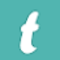## In a triangle ABC in which L is the mid point of BC and M is the mid point of AL prove that area AMC=1/4 area ABC### Text Version of the Answer is

In ∆ABC,

AL is median and cuts a triangle into two equal parts/Area

∴ ar ∆ALC = ar ALB

We know,

ar ∆ABC = ar ∆ALC + ar ∆ALB

= ar ∆ALC + ar ∆ALC

→ 1/2  ar ∆ABC = ∆ALC   ….(1)

In ∆ ALC, MC is a median

∴ a ∆AMC = Ar ∆MLC

We know,

Ar ∆ ALC = ar ∆ AMC + ar ∆MLC

1/2 ar ∆ABC = ar ∆ AMC + ar ∆AMC

(From (1))

1/2  ar ∆ ABC = 2 ar ∆ AMC

1/4  ar ∆ABC = 2 ar ∆ AMC

Hence proved.